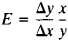# Elasticity of Consumption and Demand

The following article is from The Great Soviet Encyclopedia (1979). It might be outdated or ideologically biased.

## Elasticity of Consumption and Demand

a term used in the economic literature to denote the capacity of demand or consumption to change within certain limits under the influence of various economic factors. With respect to demand, K. Marx noted that underlying it is a social need of a given magnitude, and he pointed out the exceptional degree of elasticity of such needs. “Their fixedness is only apparent. If the means of subsistence were cheaper or if money wages were higher, the laborers would buy more of them and a greater ’social need’ would arise for them” (K. Marx and F. Engels, Soch., 2nd ed., vol. 25, parti, p. 206).

In the late 19th century the English economist A. Marshall developed a mathematical system for analyzing the dependence of demand on price and income as well as the relationship between the supply and the price of commodities. In bourgeois economics, Marshall is credited with having devised the means to determine the elasticity of consumption and demand. These ideas were subsequently developed by such economists as J. R. Hicks and P. Samuelson.

In socialist planning, it is very important to take into account the elasticity of consumption and demand. With the growth of social production and the improvement of the people’s well-being, the level and pattern of personal consumption and the retail trade turnover are constantly changing; such changes, which are influenced by various socioeconomic factors, must be taken into account in the planning of commodity production and consumption. The planning process entails the need to quantify the dependence of demand and consumption on various economic factors and to express such dependence by means of specific indexes. This dependence is expressed by the coefficient of elasticity of consumption and demand.

In Soviet economic literature, the coefficients of elasticity of consumption and demand are usually calculated on the basis of income and price; they indicate the number of percentage points by which demand (consumption) will change for every percentage point of change in these factors. If, for example, the coefficient of elasticity of demand for bread on the basis of income is 0.6, a 10-percent increase in per capita income will lead to a 6-percent increase in the population’s demand for bread.

The coefficient of elasticity of demand based on income is determined by the formulawhere e is the coefficient of elasticity, Δy is the per capita increase in demand, Δx is the per capita increase in income, x is per capita income in the base year, and y is per capita demand in the base year. As a rule, coefficients of elasticity of demand are determined on the basis of trade statistics, and coefficients of elasticity of consumption on the basis of budget statistics.

The coefficient of elasticity may also be calculated by selecting a function that graphically describes the development of the demand for a given commodity. In this case the calculation of the coefficient of elasticity is preceded by the selection and analysis of simple or multiple regression equations. Special formulas for calculating the coefficients of elasticity are derived from the most frequently used simple regression equations. For example, the formula for calculating the coefficient of elasticity of consumption and demand in the equation of the line y = a + bx is E = bx/y. Simple regression, however, reflects the influence of a single factor. In actuality, demand (consumption) is simultaneously influenced by several factors. In such cases, partial coefficients of elasticity (sometimes called net coefficients) are calculated on the basis of multiple regression equations. If, for example, the development of the demand for a given commodity is linearly dependent on income, price, and family size, the partial coefficient of elasticity of consumption and demand is calculated in turn from each individual factor, the other two factors being eliminated.

### REFERENCES

Marx, K. Kapital, vol. 3. K. Marx and F. Engels, Soch., 2nd ed., vol. 25, part 1.
Korzhenevskii, I. I. Osnovnye zakonomernosti razvitiia sprosa v SSSR, 2nd ed. Moscow, 1971.
Maslov, P. P. Izmerenie potrebitel’skogo sprosa. Moscow, 1971.
Krutikov, F. A. Izuchenie rynka tovarov narodnogo potrebleniia. Moscow, 1972.
Stolmov, L. F. Izuchenie i prognozirovanie pokupatel’skogo sprosa. Moscow, 1972.

IU. K. TVILDIANI